2019-05-20 00:41:12 qq_37367702 阅读数 210
• ###### 数据挖掘模型篇之R语言实践

理论与实践结合的方式，通过通俗易懂的教学方式培养学生运用R语言完成常用挖掘模型算法建立及评估，学习完课程可以掌握：线性回归模型、聚类分析、关联规则算法、KNN近邻算法和主成分分析等常用的模型算法实现。针对具体的数据挖掘应用需求，能熟练抽象出可合适的数据挖掘模型，并整理出其技术实现路线。

3694 人正在学习 去看看 谢佳标

1.1 knn算法概述

1.2 knn算法基本思想

1.3 knn算法方法

1.4 knn算法步骤

2.1 knn

2.2 二维示例

2.3 图像特征-稠密SIFT

2.4 图像分类：手势识别

# 一、K邻近分类法（KNN）

## 1.1 knn算法概述

K最近邻(kNN，k-NearestNeighbor)分类算法是数据挖掘分类技术中最简单的方法之一。所谓K最近邻，就是k个最近的邻居的意思，说的是每个样本都可以用它最接近的k个邻居来代表。

## 1.2 knn算法基本思想

KNN算法的基本思想是：把待分类文本表示成文本向量，与训练样本组成的样本空间中的向量计算相似度，得到k篇与该文本距离最近（最相似）的文本，根据这k篇文本所属的类别判定新文本所属的类别，在新文本的k个邻居中依次计算每类的权重，将文本分到权重最大的类中。

## 1.3 knn算法方法

• 目标：分类未知类别案例。
• 输入：待分类未知类别案例项目。已知类别案例集合D ，其中包含 j个已知类别的案例。
• 输出：项目可能的类别。

## 1.4 knn算法步骤

1. 依公式计算 Item 与 D1、D2 … …、Dj 之相似度。得到Sim(Item, D1)、Sim(Item, D2)… …、Sim(Item, Dj)。
2. 将Sim(Item, D1)、Sim(Item, D2)… …、Sim(Item, Dj)排序，若是超过相似度门槛t则放入邻居案例集合NN。
3. 自邻居案例集合NN中取出前k名，依多数决，得到Item可能类别。

# 二、实现代码及结果分析

## 2.1 knn

from numpy import *
class KnnClassifier(object):
def __init__(self,labels,samples):
""" Initialize classifier with training data. """

self.labels = labels
self.samples = samples
def classify(self,point,k=3):
""" Classify a point against k nearest
in the training data, return label. """

# compute distance to all training points
dist = array([L2dist(point,s) for s in self.samples])

# sort them
ndx = dist.argsort()

# use dictionary to store the k nearest
for i in range(k):
label = self.labels[ndx[i]]

def L2dist(p1,p2):
return sqrt( sum( (p1-p2)**2) )


## 2.2 二维示例

n = 200
# two normal distributions
class_1 = 0.7 * randn(n,2)
class_2 = 1.6 * randn(n,2) + array([6,1])
labels = hstack((ones(n),-ones(n)))
# save with Pickle
#with open('points_normal.pkl', 'wb') as f:
with open('points_normal_test.pkl', 'wb') as f:
pickle.dump(class_1,f)
pickle.dump(class_2,f)
pickle.dump(labels,f)
# normal distribution and ring around it
class_1 = 0.6 * randn(n,2)
r = 0.8 * randn(n,1) + 5
angle = 2*pi * randn(n,1)
class_2 = hstack((r*cos(angle),r*sin(angle)))
labels = hstack((ones(n),-ones(n)))
# save with Pickle
#with open('points_ring.pkl', 'wb') as f:
with open('points_ring_test.pkl', 'wb') as f:
pickle.dump(class_1,f)
pickle.dump(class_2,f)
pickle.dump(labels,f)
pklist=['points_normal.pkl','points_ring.pkl']

figure()

# load 2D points using Pickle
for i, pklfile in enumerate(pklist):
with open(pklfile, 'rb') as f:
# load test data using Pickle
with open(pklfile[:-4]+'_test.pkl', 'rb') as f:
model = knn.KnnClassifier(labels,vstack((class_1,class_2)))
# test on the first point
print (model.classify(class_1))
#define function for plotting
def classify(x,y,model=model):
return array([model.classify([xx,yy]) for (xx,yy) in zip(x,y)])
# lot the classification boundary
subplot(1,2,i+1)
imtools.plot_2D_boundary([-6,6,-6,6],[class_1,class_2],classify,[1,-1])
titlename=pklfile[:-4]
title(titlename)
show()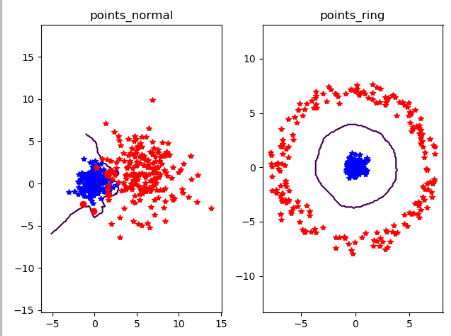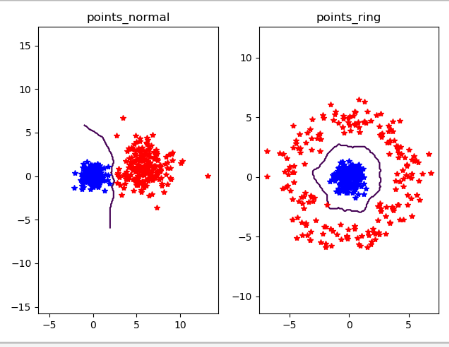## 2.3 图像特征-稠密SIFT

def process_image_dsift(imagename,resultname,size=20,steps=10,force_orientation=False,resize=None):
im = Image.open(imagename).convert('L')
if resize!=None:
im = im.resize(resize)
m,n = im.size

if imagename[-3:] != 'pgm':
#create a pgm file
im.save('tmp.pgm')
imagename = 'tmp.pgm'

# create frames and save to temporary file
scale = size/3.0
x,y = meshgrid(range(steps,m,steps),range(steps,n,steps))
xx,yy = x.flatten(),y.flatten()
frame = array([xx,yy,scale*ones(xx.shape),zeros(xx.shape)])
savetxt('tmp.frame',frame.T,fmt='%03.3f')
path = os.path.abspath(os.path.join(os.path.dirname("__file__"),os.path.pardir))
path = path + "\\ch08\\win32vlfeat\\sift.exe "
if force_orientation:
cmmd = str(path+imagename+" --output="+resultname+
else:
cmmd = str(path+imagename+" --output="+resultname+
os.system(cmmd)
print ('processed', imagename, 'to', resultname)

dsift.process_image_dsift('gesture/test/c.jpg','gesture/test/c.dsift',90,40,True)
im = array(Image.open('gesture/test/c.jpg'))
sift.plot_features(im,l,True)
title('dense SIFT')
show()

图为尚大楼，特征大小size为90，位置之间步长steps为20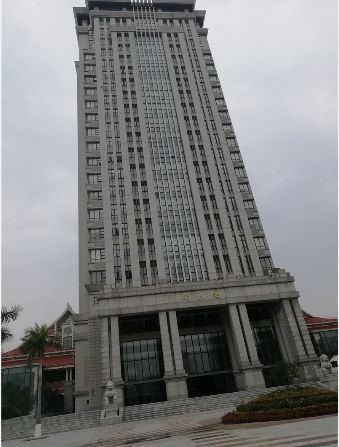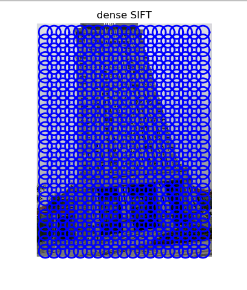## 2.4 图像分类：手势识别

def read_gesture_features_labels(path):
# create list of all files ending in .dsift
featlist = [os.path.join(path,f) for f in os.listdir(path) if f.endswith('.dsift')]
features = []
for featfile in featlist:
features.append(d.flatten())
features = array(features)
# create labels
labels = [featfile.split('/')[-1] for featfile in featlist]
return features,array(labels)

filelist_train = get_imagelist('gesture/train')
filelist_test = get_imagelist('gesture/test')
imlist=filelist_train+filelist_test

k = 1
knn_classifier = knn.KnnClassifier(labels,features)
res = array([knn_classifier.classify(test_features[i],k) for i in
range(len(test_labels))])
# accuracy
acc = sum(1.0*(res==test_labels)) / len(test_labels)
print ('Accuracy:', acc)

打印出标记及相应的混淆矩阵

def print_confusion(res,labels,classnames):
n = len(classnames)
# confusion matrix
class_ind = dict([(classnames[i],i) for i in range(n)])
confuse = zeros((n,n))
for i in range(len(test_labels)):
confuse[class_ind[res[i]],class_ind[test_labels[i]]] += 1
print ('Confusion matrix for')
print (classnames)
print (confuse)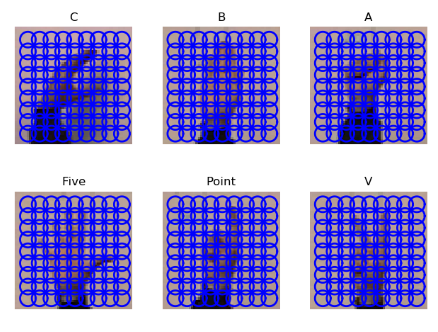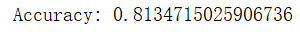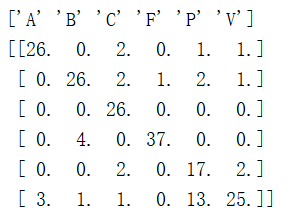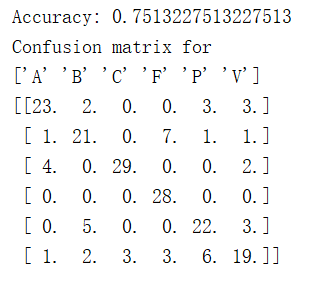knn计算机视觉 相关内容

2019-05-19 23:21:39 qq_42584188 阅读数 22
• ###### 数据挖掘模型篇之R语言实践

理论与实践结合的方式，通过通俗易懂的教学方式培养学生运用R语言完成常用挖掘模型算法建立及评估，学习完课程可以掌握：线性回归模型、聚类分析、关联规则算法、KNN近邻算法和主成分分析等常用的模型算法实现。针对具体的数据挖掘应用需求，能熟练抽象出可合适的数据挖掘模型，并整理出其技术实现路线。

3694 人正在学习 去看看 谢佳标

#### 1.KNN介绍

##### 1.1 KNN思想的基本思想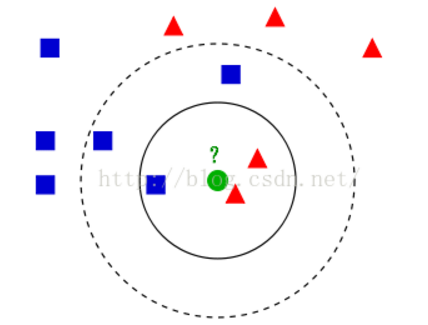##### 1.2 KNN三要素

1.距离度量
KNN算法的核心在于要找到实例点的距离。要找邻居就要度量相似性。估量不同样本之间的相似性，通常采用的方法就是计算样本间的“距离”，相似性度量方法有：欧式距离、余弦夹角、曼哈顿距离、切比雪夫距离等。
2.k值选择

3.分类决策规则

##### 1.3 KNN实现过程

（1）计算当前待分类对象与训练集中已知类标记的所有对象的距离；
（2）按照距离递增次序排序；
（3）选取与待分类对象距离最小的k个训练实例；
（4）统计这k个实例所属各个类别数；
（5）将统计的类别数最多的类别作为待分类的预测类别

#### 3.实验分析

##### 3.1 KNN的实现

class KnnClassifier(object):

def __init__(self,labels,samples):
""" Initialize classifier with training data. """
#使用训练数据初始化分类器

self.labels = labels
self.samples = samples

def classify(self,point,k=3):
""" Classify a point against k nearest
in the training data, return label. """
#在训练数据上采用k近邻分类，并返回标记

# compute distance to all training points
#计算所有训练数据点的距离
dist = array([L2dist(point,s) for s in self.samples])

# sort them
#对他们进行排序
ndx = dist.argsort()

# use dictionary to store the k nearest
#用字典存储k近邻
for i in range(k):
label = self.labels[ndx[i]]

def L2dist(p1,p2):
return sqrt( sum( (p1-p2)**2) )

##### 3.2 简单的二维示例

# -*- coding: utf-8 -*-
from numpy.random import randn
import pickle
from pylab import *

# create sample data of 2D points
n = 200
# two normal distributions
class_1 = 0.6 * randn(n,2)
class_2 = 1.2 * randn(n,2) + array([5,1])
labels = hstack((ones(n),-ones(n)))
# save with Pickle
#with open('points_normal.pkl', 'w') as f:
with open('points_normal_test.pkl', 'wb') as f:
pickle.dump(class_1,f)
pickle.dump(class_2,f)
pickle.dump(labels,f)
# normal distribution and ring around it
print ("save OK!")
class_1 = 0.6 * randn(n,2)
r = 0.8 * randn(n,1) + 5
angle = 2*pi * randn(n,1)
class_2 = hstack((r*cos(angle),r*sin(angle)))
labels = hstack((ones(n),-ones(n)))
# save with Pickle
#with open('points_ring.pkl', 'w') as f:
with open('points_ring_test.pkl', 'wb') as f:
pickle.dump(class_1,f)
pickle.dump(class_2,f)
pickle.dump(labels,f)

print ("save OK!")


# -*- coding: utf-8 -*-
import pickle
from pylab import *
from PCV.classifiers import knn
from PCV.tools import imtools

pklist=['points_normal.pkl','points_ring.pkl']

figure()

# 利用Pickle导入二维数据集
for i, pklfile in enumerate(pklist):
with open(pklfile, 'rb') as f:
# load test data using Pickle
#用Pickle模块载入测试数据
with open(pklfile[:-4]+'_test.pkl', 'rb') as f:

model = knn.KnnClassifier(labels,vstack((class_1,class_2)))
# test on the first point
#在测试数据集的第一个数据点上进行测试
print (model.classify(class_1))

#define function for plotting
#定义绘图函数
def classify(x,y,model=model):
return array([model.classify([xx,yy]) for (xx,yy) in zip(x,y)])

# lot the classification boundary
#绘制分类边界
subplot(1,2,i+1)
imtools.plot_2D_boundary([-6,6,-6,6],[class_1,class_2],classify,[1,-1])
titlename=pklfile[:-4]
title(titlename)
show()


def plot_2D_boundary(plot_range,points,decisionfcn,labels,values=):
"""    Plot_range is (xmin,xmax,ymin,ymax), points is a list
of class points, decisionfcn is a funtion to evaluate,
labels is a list of labels that decisionfcn returns for each class,
values is a list of decision contours to show. """
#Plot_range为(xmin,xmax,ymin,ymax),points是类数据点列表，decisionfcn是评估函数。
#Labels是函数decidionfcn关于每个类返回的标记列表。

#不同的类用不同颜色标记
clist = ['b','r','g','k','m','y'] # colors for the classes

# evaluate on a grid and plot contour of decision function
#在一个网格上进行评估，并画出决策函数的边界
x = arange(plot_range,plot_range,.1)
y = arange(plot_range,plot_range,.1)
xx,yy = meshgrid(x,y)
xxx,yyy = xx.flatten(),yy.flatten() # lists of x,y in grid 网格中的x，y坐标点列表
zz = array(decisionfcn(xxx,yyy))
zz = zz.reshape(xx.shape)
# plot contour(s) at values
#以values画出边界
contour(xx,yy,zz,values)

# for each class, plot the points with '*' for correct, 'o' for incorrect
#对于每类，用*画出分类正确的点，用o画出分类不正确的点
for i in range(len(points)):
d = decisionfcn(points[i][:,0],points[i][:,1])
correct_ndx = labels[i]==d
incorrect_ndx = labels[i]!=d
plot(points[i][correct_ndx,0],points[i][correct_ndx,1],'*',color=clist[i])
plot(points[i][incorrect_ndx,0],points[i][incorrect_ndx,1],'o',color=clist[i])

axis('equal')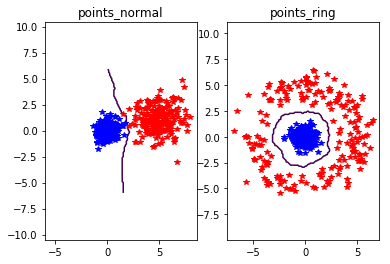r = 0.8 * randn(n,1) + 5
angle = 2*pi * randn(n,1)
class_2 = hstack((r*cos(angle),r*sin(angle)))


#左图的数据集
class_1 = 0.6 * randn(n,2)+0.6
class_2 = 1.2 * randn(n,2) + array([5,1])

#右图的数据集
class_1 = 0.6 * randn(n,2)
r = 0.8 * randn(n,1) + 2
angle = 2*pi * randn(n,1)
class_2 = hstack((r*cos(angle),r*sin(angle)))
labels = hstack((ones(n),-ones(n)))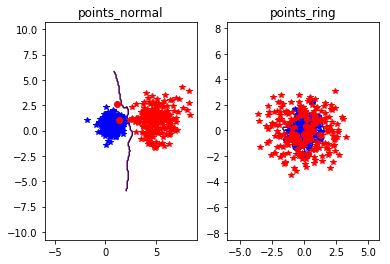k值选择

##### 3.3 图像分类：手势识别

# -*- coding: utf-8 -*-
import os
from PCV.localdescriptors import sift, dsift
from pylab import  *
from PIL import Image

imlist=['gesture/train/C-uniform02.ppm','gesture/train/B-uniform01.ppm',
'gesture/train/A-uniform01.ppm','gesture/train/Five-uniform01.ppm',
'gesture/train/Point-uniform01.ppm','gesture/train/V-uniform01.ppm']

figure()
for i, im in enumerate(imlist):
print (im)
dsift.process_image_dsift(im,im[:-3]+'dsift',11,5,True)
dirpath, filename=os.path.split(im)
im = array(Image.open(im))
#显示手势含义title
titlename=filename[:-14]
subplot(2,3,i+1)
sift.plot_features(im,l,True)
title(titlename)
show()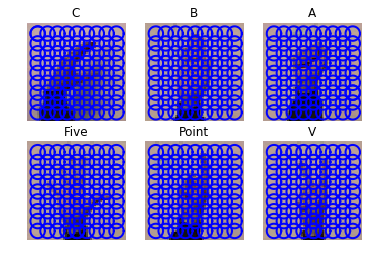import os
from PCV.localdescriptors import sift, dsift
from PCV.tools import imtools
from pylab import  *
from PIL import Image

imlist = imtools.get_imlist('gesture/train')
for filename in imlist:
featfile = filename[:-3]+'dsift'
dsift.process_image_dsift(filename,featfile,10,5,resize=(50,50));


# -*- coding: utf-8 -*-
from PCV.localdescriptors import dsift
import os
from PCV.localdescriptors import sift
from pylab import *
from PCV.classifiers import knn

def get_imagelist(path):
"""    Returns a list of filenames for
all jpg images in a directory. """

return [os.path.join(path,f) for f in os.listdir(path) if f.endswith('.jpg')]

# 多所有以.dsift为后缀的文件创建一个列表
featlist = [os.path.join(path,f) for f in os.listdir(path) if f.endswith('.dsift')]
# 读取特征
features = []
for featfile in featlist:
features.append(d.flatten())
features = array(features)
# 创建标记
labels = [featfile.split('/')[-1] for featfile in featlist]
return features,array(labels)

def print_confusion(res,labels,classnames):
n = len(classnames)
# 混淆矩阵
class_ind = dict([(classnames[i],i) for i in range(n)])
confuse = zeros((n,n))
for i in range(len(test_labels)):
confuse[class_ind[res[i]],class_ind[test_labels[i]]] += 1
print ('Confusion matrix for')
print (classnames)
print (confuse)

filelist_train = get_imagelist('gesture/train')
filelist_test = get_imagelist('gesture/test')
imlist=filelist_train+filelist_test

# process images at fixed size (50,50)
for filename in imlist:
featfile = filename[:-3]+'dsift'
dsift.process_image_dsift(filename,featfile,10,5,resize=(50,50))

classnames = unique(labels)

# 测试 kNN
k = 1
knn_classifier = knn.KnnClassifier(labels,features)
res = array([knn_classifier.classify(test_features[i],k) for i in
range(len(test_labels))])
# 准确率
acc = sum(1.0*(res==test_labels)) / len(test_labels)
print ('Accuracy:', acc)

print_confusion(res,test_labels,classnames)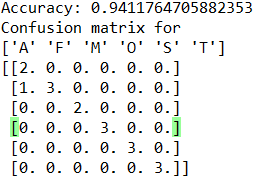k值对分类的影响
1.该结果是在k=1，以及稠密SIFT图像描述子的大小为10，位置之间的步长为5的情况下的结果，正确率为94.12%，Four的有张手势照片出错。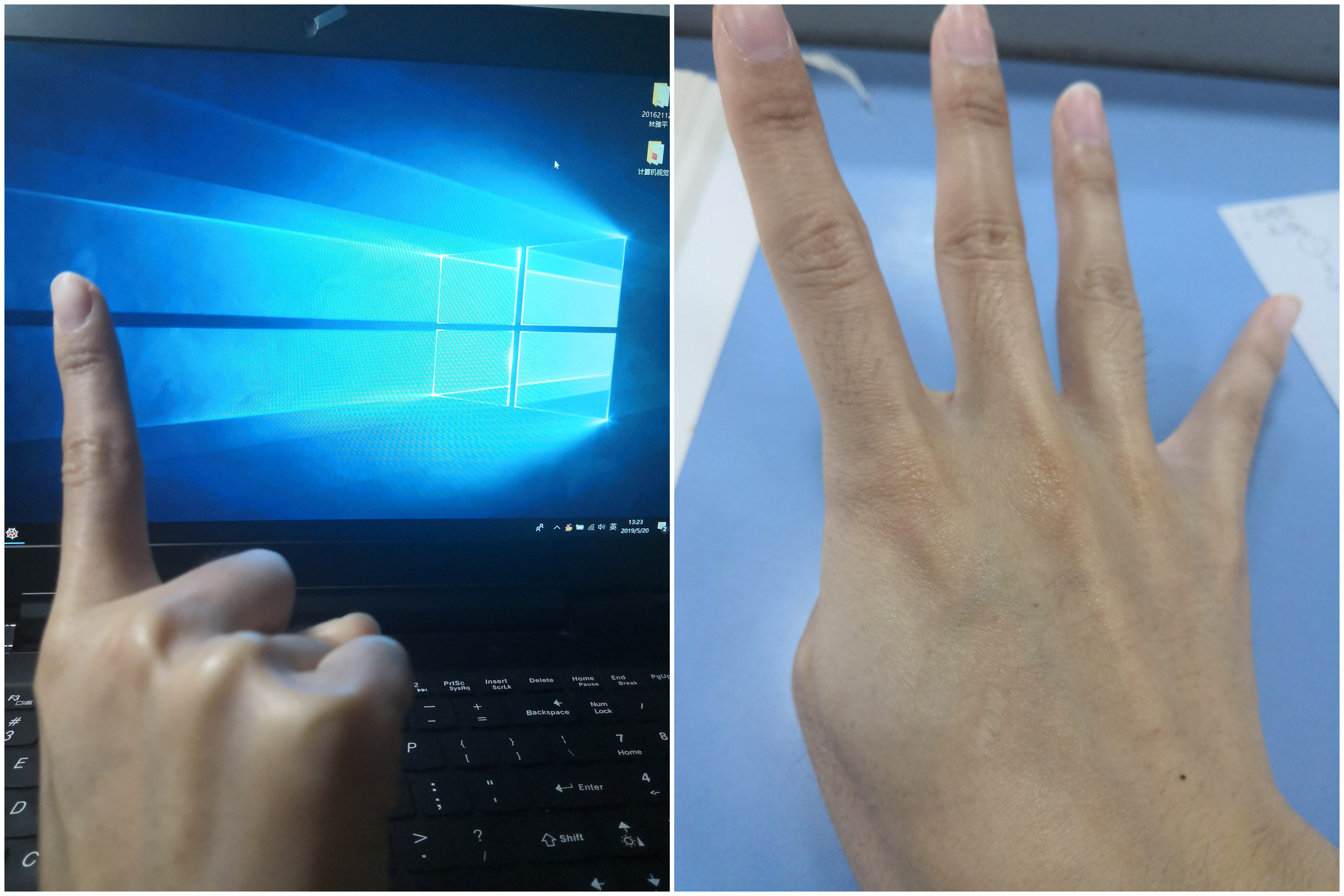2.下图结果是在k=3，以及稠密SIFT图像描述子的大小为10，位置之间的步长为5的情况下的结果，正确率为88.23%。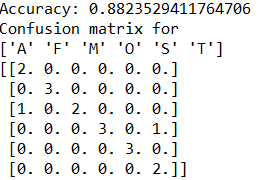3.下图结果是在k=6，以及稠密SIFT图像描述子的大小为10，位置之间的步长为5的情况下的结果，正确率为76.47%。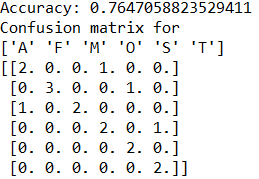1.下图结果是在稠密SIFT图像描述子的大小为10，位置之间的步长为5，以及k=1的情况下的结果，正确率为94.12%，2.下图结果是在稠密SIFT图像描述子的大小为5，位置之间的步长为5，以及k=1的情况下的结果，正确率为94.12%，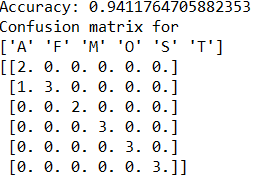3.下图结果是在稠密SIFT图像描述子的大小为2，位置之间的步长为5，以及k=1的情况下的结果，正确率为94.12%，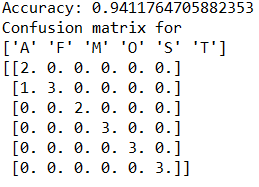4.下图结果是在稠密SIFT图像描述子的大小为15，位置之间的步长为5，以及k=1的情况下的结果，正确率为94.12%，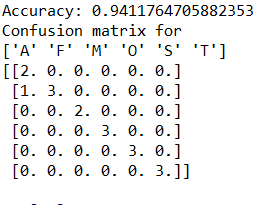5.下图结果是在稠密SIFT图像描述子的大小为25，位置之间的步长为5，以及k=1的情况下的结果，正确率为94.12%，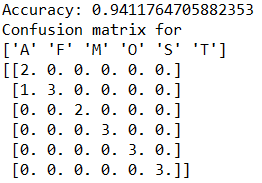1） 1，2，3的实验是描述子的大小逐渐减小，正确率没有改变。4到5是描述子的增大，正确率没有改变。
5.下图结果是在稠密SIFT图像描述子的大小为25，位置之间的步长为5，以及k=6的情况下的结果，正确率为82.36%.我们在前面的实验曾经测试过在“k=6，以及稠密SIFT图像描述子的大小为10，位置之间的步长为5的情况下的结果，正确率为76.47%”。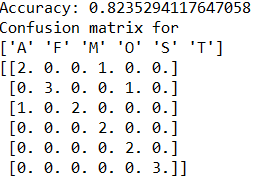6.下图结果是在稠密SIFT图像描述子的大小为5，位置之间的步长为5，以及k=6的情况下的结果，正确率为82.36%.我们在前面的实验曾经测试过在“k=6，以及稠密SIFT图像描述子的大小为10，位置之间的步长为5的情况下的结果，正确率为76.47%”。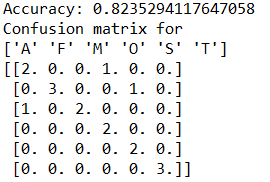2） 在相同步长的情况下，描述子小的话，每个特征所包含的信息会少，相反大的话，每个特征所包含的信息多。但在实验中我们需要的最好的描述子的大小，是能够确保每个描述子能够将我们所需要的信息都包含进去。综上实验表明，特征描述子的大小在分类器的k值非常适合时，可能不会再影响到实验的结果，不会让实验结果变得更好，也不会更糟糕，但在k值不是那么合适的时候，特征描述子的大小会影响到实验结果，从上面的5跟6可以看出特征描述子不管变小还是变大，在k值不合适的时候都会增加分类的正确性。

knn计算机视觉 相关内容

2016-06-08 20:59:30 littlethunder 阅读数 20628
• ###### 数据挖掘模型篇之R语言实践

理论与实践结合的方式，通过通俗易懂的教学方式培养学生运用R语言完成常用挖掘模型算法建立及评估，学习完课程可以掌握：线性回归模型、聚类分析、关联规则算法、KNN近邻算法和主成分分析等常用的模型算法实现。针对具体的数据挖掘应用需求，能熟练抽象出可合适的数据挖掘模型，并整理出其技术实现路线。

3694 人正在学习 去看看 谢佳标

OCR应用非常广泛，而且有许多方法，今天用KNN算法实现简单的0-9手写数字识别。本程序使用OpenCV 3.0和Python 3。

KNN算法是K近邻分类算法，属于机器学习中的监督学习，需要一定量的带标签的输入样本数据进行“训练”，然后就可以识别。我给“训练”打引号是因为其实KNN没有明显的前期训练过程，它是要给一个样本x分类，就从数据集中在x附近找离它最近的k各数据点，这k个数据点中包含的y类别最多，那么就把x的标签标记为y，这就完成了分类识别的过程。def initKnn():
knn = cv2.ml.KNearest_create()
gray = cv2.cvtColor(img, cv2.COLOR_BGR2GRAY)
cells = [np.hsplit(row,100) for row in np.vsplit(gray,50)]
train = np.array(cells).reshape(-1,400).astype(np.float32)
trainLabel = np.repeat(np.arange(10),500)
return knn, train, trainLabel


def updateKnn(knn, train, trainLabel, newData=None, newDataLabel=None):
if newData != None and newDataLabel != None:
print(train.shape, newData.shape)
newData = newData.reshape(-1,400).astype(np.float32)
train = np.vstack((train,newData))
trainLabel = np.hstack((trainLabel,newDataLabel))
knn.train(train,cv2.ml.ROW_SAMPLE,trainLabel)
return knn, train, trainLabel

updateKnn是增加自己的训练数据后更新Knn的操作。

def findRoi(frame, thresValue):
rois = []
gray = cv2.cvtColor(frame, cv2.COLOR_BGR2GRAY)
gray2 = cv2.dilate(gray,None,iterations=2)
gray2 = cv2.erode(gray2,None,iterations=2)
edges = cv2.absdiff(gray,gray2)
x = cv2.Sobel(edges,cv2.CV_16S,1,0)
y = cv2.Sobel(edges,cv2.CV_16S,0,1)
absX = cv2.convertScaleAbs(x)
absY = cv2.convertScaleAbs(y)
ret, ddst = cv2.threshold(dst,thresValue,255,cv2.THRESH_BINARY)
im, contours, hierarchy = cv2.findContours(ddst,cv2.RETR_EXTERNAL, cv2.CHAIN_APPROX_SIMPLE)
for c in contours:
x, y, w, h = cv2.boundingRect(c)
if w > 10 and h > 20:
rois.append((x,y,w,h))
return rois, edges

findRoi函数是找到每个数字的位置，用包裹其最小矩形的左上顶点的坐标和该矩形长宽表示(x, y, w, h)。这里还用到了Sobel算子。edges是原始图像形态变换之后的灰度图，可以排除一些背景的影响，比如本子边缘、纸面的格子、手、笔以及影子等等，用edges来获取数字图像效果比Sobel获取的边界效果要好。

def findDigit(knn, roi, thresValue):
ret, th = cv2.threshold(roi, thresValue, 255, cv2.THRESH_BINARY)
th = cv2.resize(th,(20,20))
out = th.reshape(-1,400).astype(np.float32)
ret, result, neighbours, dist = knn.findNearest(out, k=5)
return int(result), th

findDigit函数是用KNN来分类，并将结果返回。th是用来手动输入训练数据时显示的图片。20x20pixel的尺寸是OpenCV自带digits.png中图像尺寸，因为我是在其基础上更新数据，所以沿用这个尺寸。

def concatenate(images):
n = len(images)
output = np.zeros(20*20*n).reshape(-1,20)
for i in range(n):
output[20*i:20*(i+1),:] = images[i]
return output

concatenate函数是拼接数字图像并显示的，用来输入训练数据。

while True:
frame = frame[:,:426]
rois, edges = findRoi(frame, 50)
digits = []
for r in rois:
x, y, w, h = r
digit, th = findDigit(knn, edges[y:y+h,x:x+w], 50)
digits.append(cv2.resize(th,(20,20)))
cv2.rectangle(frame, (x,y), (x+w,y+h), (153,153,0), 2)
cv2.putText(frame, str(digit), (x,y), cv2.FONT_HERSHEY_SIMPLEX, 1, (127,0,255), 2)
newEdges = cv2.cvtColor(edges, cv2.COLOR_GRAY2BGR)
newFrame = np.hstack((frame,newEdges))
cv2.imshow('frame', newFrame)
videoFrame.write(newFrame)
key = cv2.waitKey(1) & 0xff
if key == ord(' '):
break
elif key == ord('x'):
Nd = len(digits)
output = concatenate(digits)
showDigits = cv2.resize(output,(60,60*Nd))
cv2.imshow('digits', showDigits)
cv2.imwrite(str(count)+'.png', showDigits)
count += 1
if cv2.waitKey(0) & 0xff == ord('e'):
pass
print('input the digits(separate by space):')
numbers = input().split(' ')
Nn = len(numbers)
if Nd != Nn:
print('update KNN fail!')
continue
try:
for i in range(Nn):
numbers[i] = int(numbers[i])
except:
continue
knn, train, trainLabel = updateKnn(knn, train, trainLabel, output, numbers)
print('update KNN, Done!')knn计算机视觉 相关内容

2015-11-20 17:16:17 yaoqiang2011 阅读数 47306
• ###### 数据挖掘模型篇之R语言实践

理论与实践结合的方式，通过通俗易懂的教学方式培养学生运用R语言完成常用挖掘模型算法建立及评估，学习完课程可以掌握：线性回归模型、聚类分析、关联规则算法、KNN近邻算法和主成分分析等常用的模型算法实现。针对具体的数据挖掘应用需求，能熟练抽象出可合适的数据挖掘模型，并整理出其技术实现路线。

3694 人正在学习 去看看 谢佳标

## 1. 图像分类问题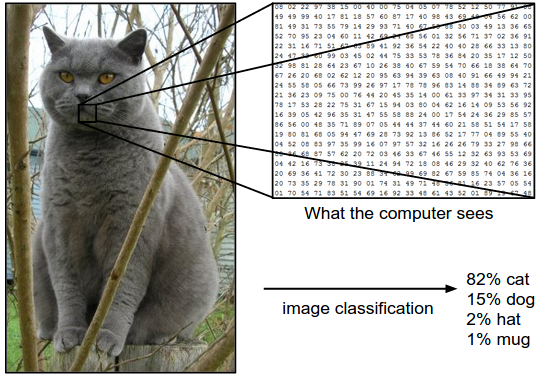### 1.1 图像识别的难点

• 视角不同，每个事物旋转或者侧视最后的构图都完全不同
• 尺寸大小不统一，相同内容的图片也可大可小
• 变形，很多东西处于特殊的情形下，会有特殊的摆放和形状
• 光影等干扰/幻象
• 背景干扰
• 同类内的差异(比如椅子有靠椅/吧椅/餐椅/躺椅…)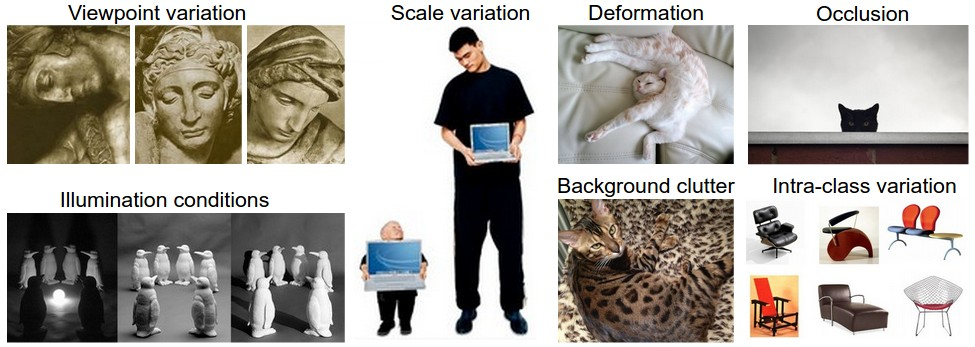### 1.2 识别的途径### 1.3 机器学习解决图像分类的流程/Pipeline

• 输入：我们的给定K个类别的N张图片，作为计算机学习的训练集
• 学习：让计算机逐张图片地『观察』和『学习』
• 评估：就像我们上学学了东西要考试检测一样，我们也得考考计算机学得如何，于是我们给定一些计算机不知道类别的图片让它判别，然后再比对我们已知的正确答案。

## 2. 最近邻分类器(Nearest Neighbor Classifier)

### 2.1 CIFAR-10

CIFAR-10是一个非常常用的图像分类数据集。数据集包含60000张32*32像素的小图片，每张图片都有一个类别标注(总共有10类)，分成了50000张的训练集和10000张的测试集。如下是一些图片示例：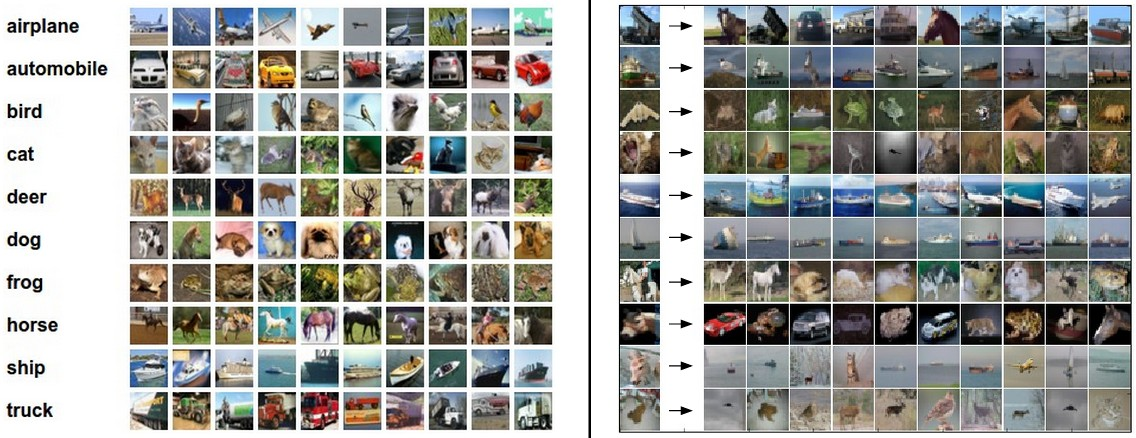### 2.2 基于最近邻的简单图像类别判定

d1(I1,I2)=pIp1Ip2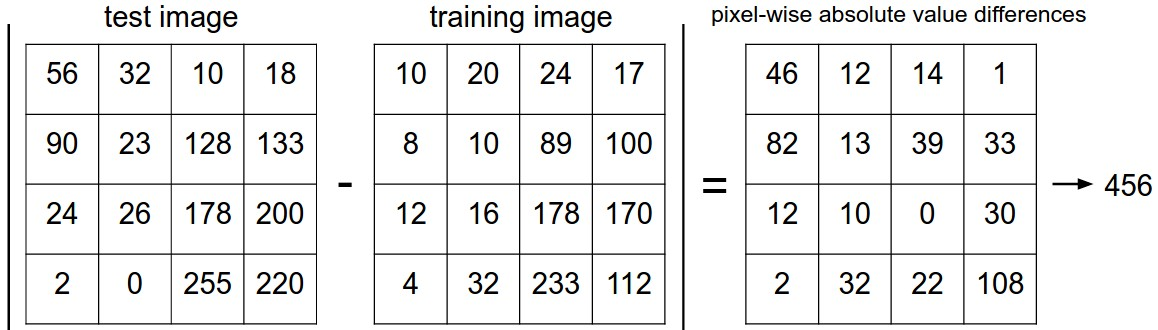#! /usr/bin/env python
#coding=utf-8
import os
import sys
import numpy as np

"""
cifar-10数据集是分batch存储的，这是载入单个batch

@参数 filename: cifar文件名
@r返回值: X, Y: cifar batch中的 data 和 labels
"""

with open(filename, 'r') as f:

X=X.reshape(10000, 3, 32, 32).transpose(0,2,3,1).astype("float")
Y=np.array(Y)

return X, Y

"""
读取载入整个 CIFAR-10 数据集

@参数 ROOT: 根目录名
@return: X_train, Y_train: 训练集 data 和 labels
X_test, Y_test: 测试集 data 和 labels
"""

xs=[]
ys=[]

for b in range(1,6):
f=os.path.join(ROOT, "data_batch_%d" % (b, ))
xs.append(X)
ys.append(Y)

X_train=np.concatenate(xs)
Y_train=np.concatenate(ys)

del X, Y

return X_train, Y_train, X_test, Y_test

# 载入训练和测试数据集
X_train, Y_train, X_test, Y_test = load_CIFAR10('data/cifar10/')
# 把32*32*3的多维数组展平
Xtr_rows = X_train.reshape(X_train.shape, 32 * 32 * 3) # Xtr_rows : 50000 x 3072
Xte_rows = X_test.reshape(X_test.shape, 32 * 32 * 3) # Xte_rows : 10000 x 3072

class NearestNeighbor:
def __init__(self):
pass

def train(self, X, y):
"""
这个地方的训练其实就是把所有的已有图片读取进来 -_-||
"""
# the nearest neighbor classifier simply remembers all the training data
self.Xtr = X
self.ytr = y

def predict(self, X):
"""
所谓的预测过程其实就是扫描所有训练集中的图片，计算距离，取最小的距离对应图片的类目
"""
num_test = X.shape
# 要保证维度一致哦
Ypred = np.zeros(num_test, dtype = self.ytr.dtype)

# 把训练集扫一遍 -_-||
for i in xrange(num_test):
# 计算l1距离，并找到最近的图片
distances = np.sum(np.abs(self.Xtr - X[i,:]), axis = 1)
min_index = np.argmin(distances) # 取最近图片的下标
Ypred[i] = self.ytr[min_index] # 记录下label

return Ypred

nn = NearestNeighbor() # 初始化一个最近邻对象
nn.train(Xtr_rows, Y_train) # 训练...其实就是读取训练集
Yte_predict = nn.predict(Xte_rows) # 预测
# 比对标准答案，计算准确率
print 'accuracy: %f' % ( np.mean(Yte_predict == Y_test) )

### 2.3 关于最近邻的距离准则

• 比如说l2距离(也就是大家熟知的欧氏距离)的计算准则如下：

d2(I1,I2)=p(Ip1Ip2)2

• 比如余弦距离计算准则如下：

1I1I2||I1||||I2||

## 3. K最近邻分类器(K Nearest Neighbor Classifier)

KNN其实是一种特别常用的分类算法。但是有个问题，我们的K值应该取多少呢。换句话说，我们找多少邻居来投票，比较靠谱呢？

### 3.1 交叉验证与参数选择

• 距离的选择(l1,l2,cos等等)
• 近邻个数K的取值。

# 假定已经有Xtr_rows, Ytr, Xte_rows, Yte了，其中Xtr_rows为50000*3072 矩阵
Xval_rows = Xtr_rows[:1000, :] # 构建1000的交叉验证集
Yval = Ytr[:1000]
Xtr_rows = Xtr_rows[1000:, :] # 保留49000的训练集
Ytr = Ytr[1000:]

# 设置一些k值，用于试验
validation_accuracies = []
for k in [1, 3, 5, 7, 10, 20, 50, 100]:

# 初始化对象
nn = NearestNeighbor()
nn.train(Xtr_rows, Ytr)
# 修改一下predict函数，接受 k 作为参数
Yval_predict = nn.predict(Xval_rows, k = k)
acc = np.mean(Yval_predict == Yval)
print 'accuracy: %f' % (acc,)

# 输出结果
validation_accuracies.append((k, acc))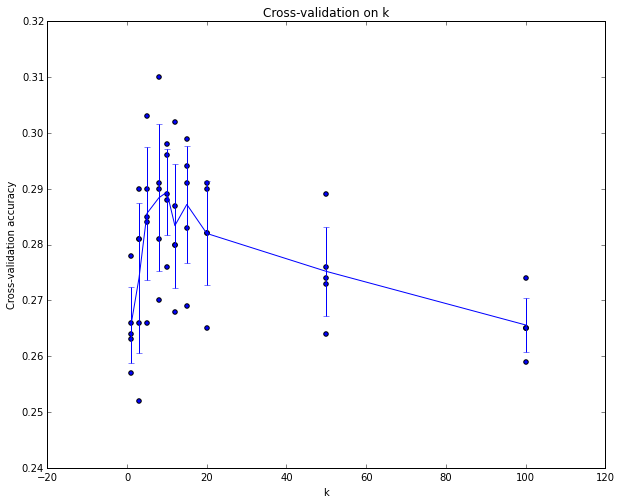### 3.2 最近邻方法的优缺点

K最近邻的优点大家都看出来了，思路非常简单清晰，而且完全不需要训练…不过也正因为如此，最后的predict过程非常耗时，因为要和全部训练集中的图片比对一遍。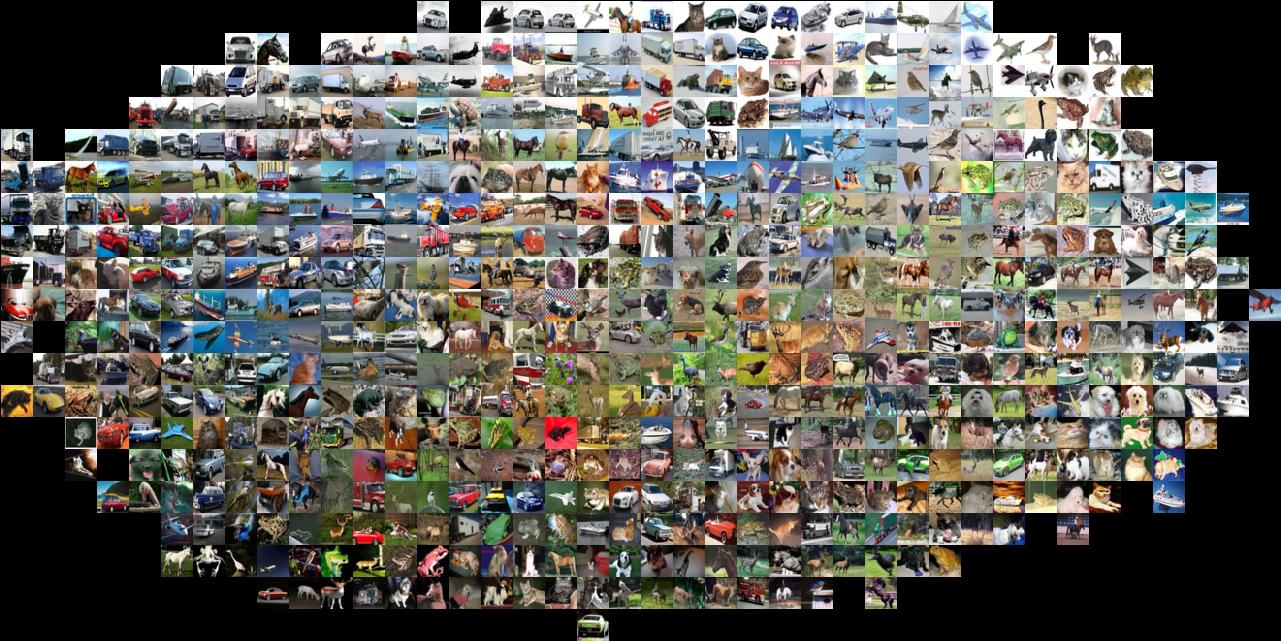## 参考资料与原文

cs231n 图像分类与KNN

knn计算机视觉 相关内容

2018-10-15 13:49:58 Sousky 阅读数 112
• ###### 数据挖掘模型篇之R语言实践

理论与实践结合的方式，通过通俗易懂的教学方式培养学生运用R语言完成常用挖掘模型算法建立及评估，学习完课程可以掌握：线性回归模型、聚类分析、关联规则算法、KNN近邻算法和主成分分析等常用的模型算法实现。针对具体的数据挖掘应用需求，能熟练抽象出可合适的数据挖掘模型，并整理出其技术实现路线。

3694 人正在学习 去看看 谢佳标

### week 1  10/11-10/14

-->为什么要有验证集

-->交叉验证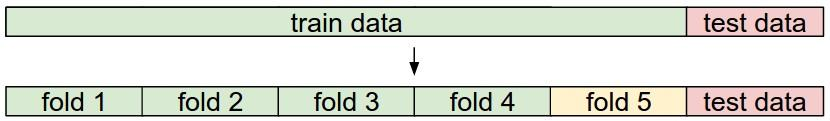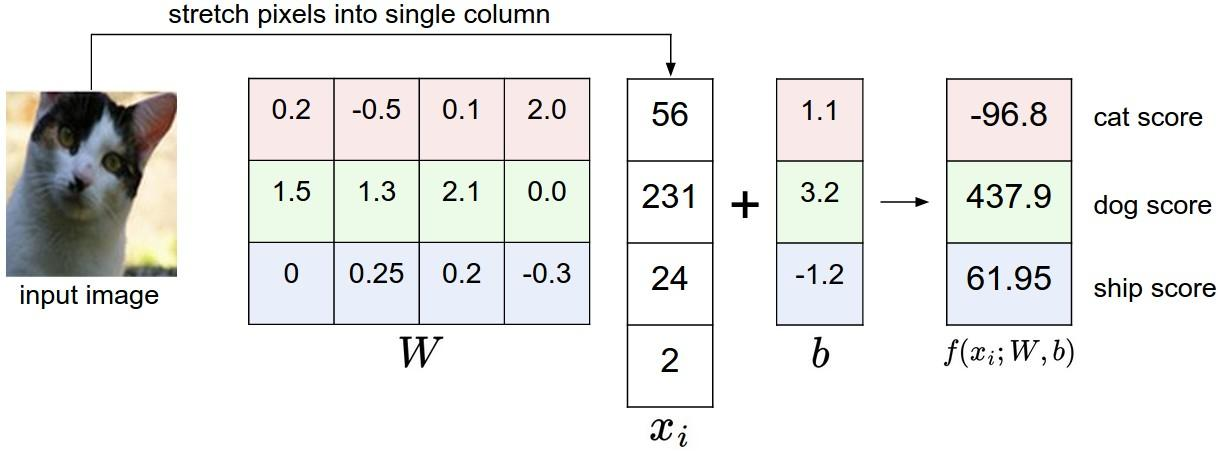-->即W中一行代表一个类别，有多少类，W就有多少行。每一行点乘像素加上偏置就是每类别的分数。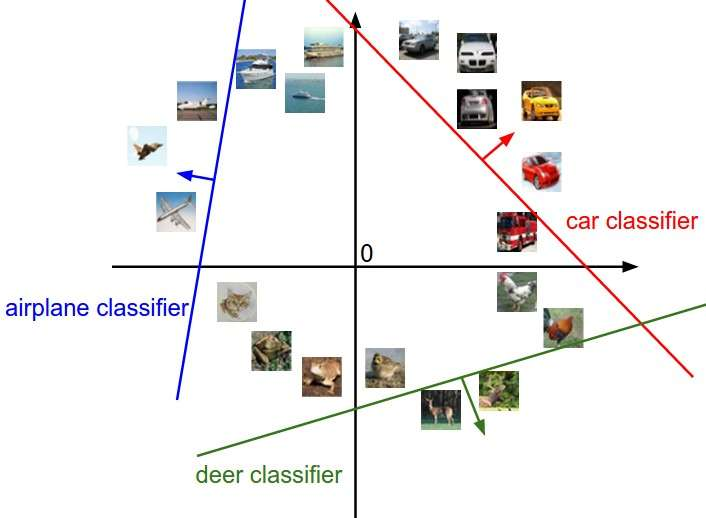1.阅读python和numpy教程（链接：https://zhuanlan.zhihu.com/p/20878530?refer=intelligentunit ）和代码（https://github.com/sharedeeply/cs231n-camp/blob/master/tutorial/python_numpy_tutorial.ipynb ）写一个矩阵的类，实现矩阵乘法，只能使用 python 的类(class)和列表(list)。

2. 完成assignment1中的knn.ipynb

knn计算机视觉 相关内容